# Right triangle - 6th grade (11y) - math problems

1. Chauncey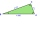Chauncey is building a storage bench for his son’s playroom. The storage bench will fit into the corner and against two walls to form a triangle. Chanuncy wants to buy a triangular shaped cover for the bench. If the storage bench is 2 1/2 ft. Along one w
2. Triangular prismCalculate a triangular prism if it has a rectangular triangle base with a = 4cm and hypotenuse c = 50mm and height of the prism is 0.12 dm.
3. Perpendicular prism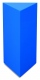Calculate the volume of the perpendicular prism if its height is 60.8 cm and the base is a rectangular triangle with 40.4 cm and 43 cm legs.
4. Display case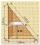Place a glass shelf at the height of 1m from the bottom of the display case in the cabinet. How long platter will we place at this height? The display case is a rectangular triangle with 2 m and 2.5 m legs.
5. Area of RT 2Calculate the area of right triangle whose legs have a length 5.8 cm and 5.8 cm.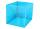I have homework. The cube has an edge 7 cm long and I must find wall and body diagonal.
7. SimilarityAre two right triangles similar to each other if the first one has a acute angle 70° and second one has acute angle 20°?
8. It is rectangular?Size of two of the angles in a triangle are: α=110°, β=40°. Is it a right triangle?
9. TriangleCan be rectangular triangle equilateral?
10. Triangle P2Can triangle have two right angles?
11. Right triangleRight triangle legs has lengths 630 mm and 411 dm. Calculate the area of this triangle.
12. Rectangular trapezoid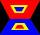How many inner right angles has a rectangular trapezoid?

We apologize, but in this category are not a lot of examples.
Do you have an interesting mathematical word problem that you can't solve it? Enter it, and we can try to solve it.

We will send a solution to your e-mail address. Solved examples are also published here. Please enter the e-mail correctly and check whether you don't have a full mailbox.

Please do not submit problems from current active competitions such as Mathematical Olympiad, correspondence seminars etc...